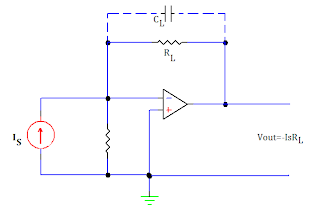# Voltage and Current Converter Using OP AMP

## VOLTAGE TO CURRENT CONVERTER USING OP AMP

Occasionally in industrial electronics, it is necessary to provide current proportional to certain voltage, even though the load resistance may vary. A circuit which can perform this job, is called a Voltage to current converter. The circuit is similar to that of positive scalar except that the feedback resistor Rf is replaced by a load resistor RL. For a single input, the current in the load resistor is given by IL = I = Vin/R. from this equation it is obvious that the output current IL is independent of load resistance and is proportional to the input voltage. This is because of the virtual ground at the inverting input terminal of the op-amp. Such a circuit is employed in analog-to-digital converter (ADC).Voltage to Current Converter

One good thing with the op amp voltage to current converter is that it can be driven by a voltage source which is itself not capable of supplying the load current. This is because the voltage source only has to drive a noninverting operational amplifier, whose input impedance is very high. The load current itself is supplied by the op amp.
Another version of voltage to current converter is given below:-

In this circuit, the load is grounded, and an input voltage controls the load current.

## CURRENT TO VOLTAGE CONVERTERS USING OP AMPCurrent Converter

A device that produces a voltage proportional to the input signal current is called a current to voltage converter. In this circuit, a photocell or photo multiplier tube that provides output current proportional to the light flux but independent of the load impedance, is connected to the inverting input terminal of the op-amp. Rs is the shunt source resistance, as there is a virtual ground at the inverting input terminal, current flowing through Rs is zero and, therefore, the entire current Is flows through the load resistor RL resulting in the output voltage given as:- Vout = -IsRL
The above equation clearly indicates that the output voltage is directly proportional to the input (source) current Is. However, in this circuit, the lower limit on current measurement is set by the bias current of the inverting input. Often a capacitor CL is connected in parallel with resistor RL for reducing the high frequency noise.
One of the most important uses of current to voltage converter is in sensing from photo-detectors and in digital to analog converter applications### Tittu

#### Author & Editor

I'm Tittu Thomas, An Electronics and Communication Engineer from India, Kerala. I love doing hobby electronics circuits, blogging, programming, etc. Started this blog while doing my B-Tech degree under CUSAT university.